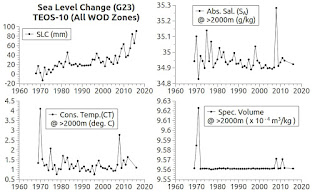## Monday, July 9, 2018

### The World According To Measurements - 15Fig. 1Fig. 2Fig. 3
In a recent post that shows (with source code provided) the unique nature of water, I wrote:
"I am going to expand this software logic to the WOD in situ measurements database so as to see how it applies in different parts of the world ocean."
(Proof of Concept - 10). Today I am showing the results of accomplishing that task.

I use the TEOS-10 library functions to accomplish another way to detect volume change.

Originally I had used the general formula: "ΔV = V0 β ΔT or V1 = V0 * β * (T0 - T1)" to calculate thermosteric volume changes (Golden 23 Zones Meet TEOS-10).

That formula has advantages in that it relates to a large body of seawater.

One disadvantage is that it is not a TEOS-10 formula, but it is a common formula for calculating volume change due to temperature change.

Further, all of the variables in it are calculated using TEOS-10 functions (ibid).

Today's graphs are generated completely with values computed using only TEOS-10 functions (except for the PSMSL tide gauge pane on each graph).

The function  gsw_specvol (SA, CT, P) calculates volume changes in a cubic meter of seawater ( m3 / kg ) using TEOS-10 values: Absolute Salinity (SA), Conservative Temperature (CT), and pressure (P).

Those TEOS-10 values are calculated from the in situ WOD Measurements recorded in their vast database.

Three depth-layer depictions are made using data from 0-700 m (Fig. 1), >700m - 2000m (Fig. 2), and >2000 m (Fig. 3).

(Note that "m" means meters.)

The take-home is that once again the measurements show that all the fresh water entering the ocean from the melting of the vast ice sheets is impacting temperatures, salinities, and volume at different amounts at different depth levels.

Today's graphs use all WOD in situ measurements, so, in the future I may add graphs of the hemispheres, the layers, and perhaps even individual zones.

Stay tuned.

The next post in this series is here, the previous post in this series is here.